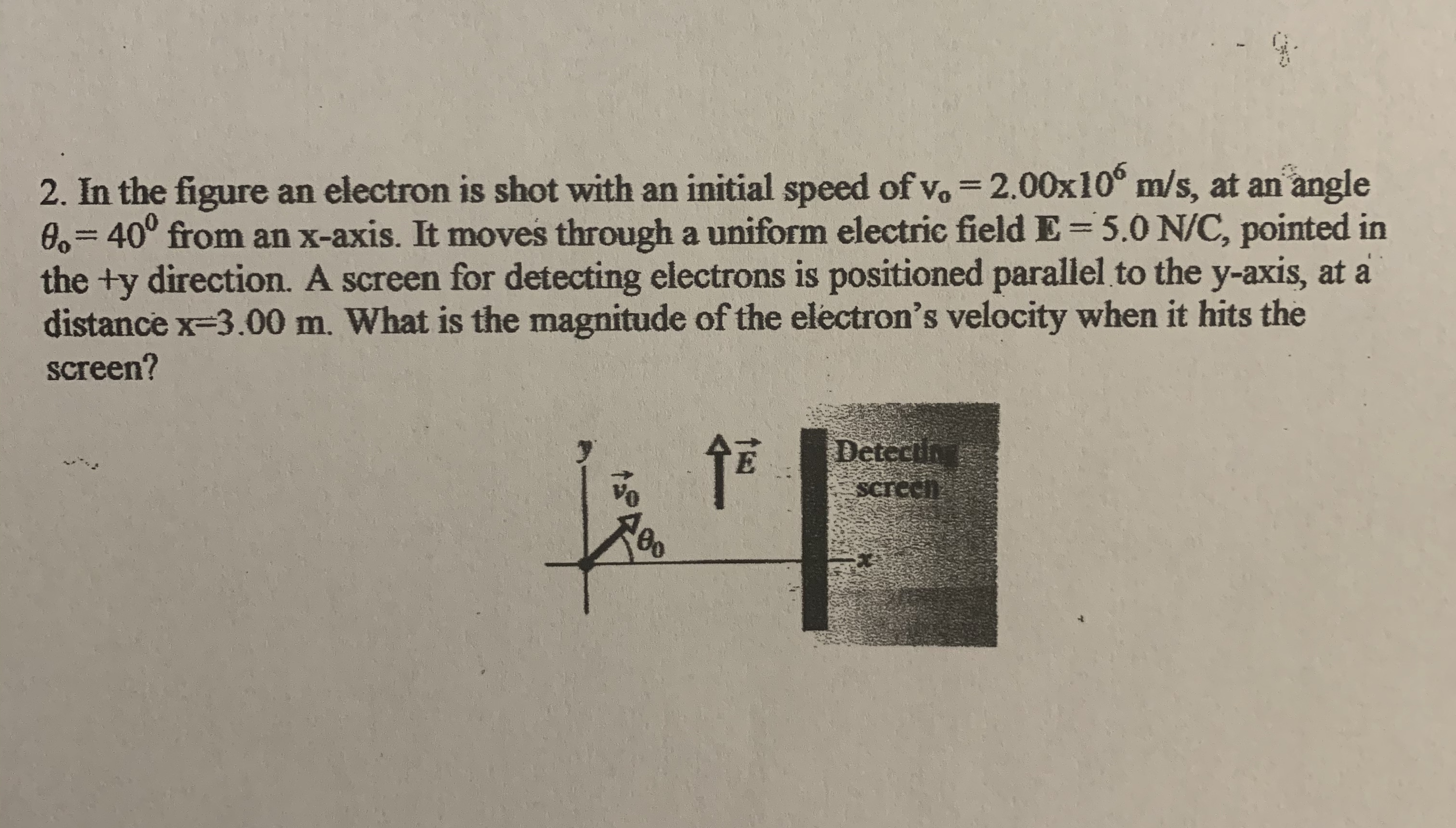# 2. In the figure an electron is shot with an initial speed of vo = 2.00x10° m/s, at an angle0,340° from an x-axis. It moves through a uniform electric field E=5.0 N/C, pointed inthe +y direction. A screen for detecting electrons is positioned parallel to the y-axis, at adistance x-3.00 m. What is the magnitude of the electron's velocity when it hits thescreen?%3DDetectinScreeh

Question
12 viewshelp_outlineImage Transcriptionclose2. In the figure an electron is shot with an initial speed of vo = 2.00x10° m/s, at an angle 0,340° from an x-axis. It moves through a uniform electric field E=5.0 N/C, pointed in the +y direction. A screen for detecting electrons is positioned parallel to the y-axis, at a distance x-3.00 m. What is the magnitude of the electron's velocity when it hits the screen? %3D Detectin Screeh fullscreen
check_circle

Step 1

Given

Initial velocity Vo =2.00 x 106 m/s

Angle θo = 40o

Electric field E = 5.0 N/C

Screen distance X = 3.00 m

Known values

Charge of electron q = 1.6 x10-19 C

Mass of electron m = 9.12 x 10-31 kg

Step 2

Solution

Resolving the initial velocity of the electron into X and Y components

Step 3

Since the field is acting only in y direction, there is no acceleration in x direction. So the final velocity in x dir...

### Want to see the full answer?

See Solution

#### Want to see this answer and more?

Solutions are written by subject experts who are available 24/7. Questions are typically answered within 1 hour.*

See Solution
*Response times may vary by subject and question.
Tagged in

### Electric Charges and Fields